Question

A film distribution manager calculates that 9% of the films released are flops. If the manager is accurate, what is the probability that the proportion of flops in a sample of 517 released films would differ from the population proportion by more than 3%? Round your answer to four decimal places.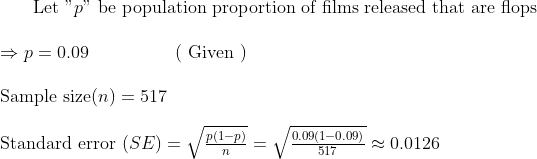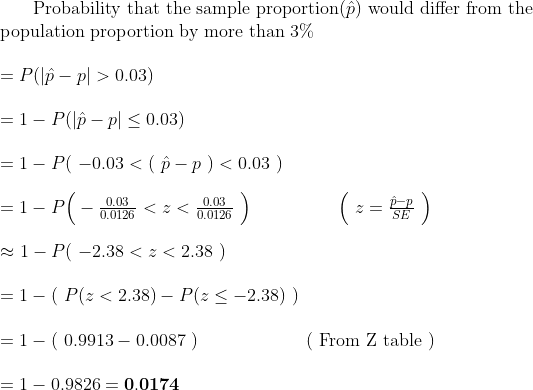#### Earn Coins

Coins can be redeemed for fabulous gifts.

Similar Homework Help Questions
• ### A film distribution manager calculates that 9% of the films released are flops. If the manager...

A film distribution manager calculates that 9% of the films released are flops. If the manager is correct, what is the probability that the proportion of flops in a sample of 407 released films would differ from the population proportion by more than 3%? Round your answer to four decimal places. Answer: 0.0348

• ### A film distribution manager calculates that 6 % of the films released are flops. If the...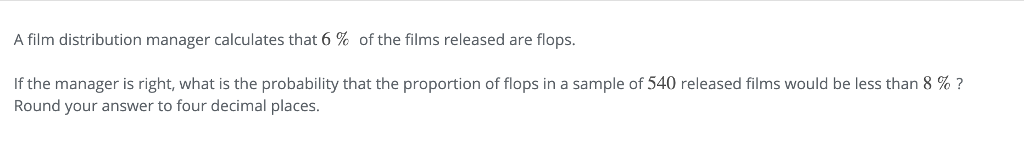A film distribution manager calculates that 6 % of the films released are flops. If the manager is right, what is the probability that the proportion of flops in a sample of 540 released films would be less than 8 % ? Round your answer to four decimal places.

• ### A film distribution manager calculates that 7%of the films released are flops. If the manager is...

A film distribution manager calculates that 7%of the films released are flops. If the manager is correct, what is the probability that the proportion of flops in a sample of 653 released films would be less than 6% ? Round your answer to four decimal places.

• ### Correct A film distribution manager calculates that of the films released are flops if the manager...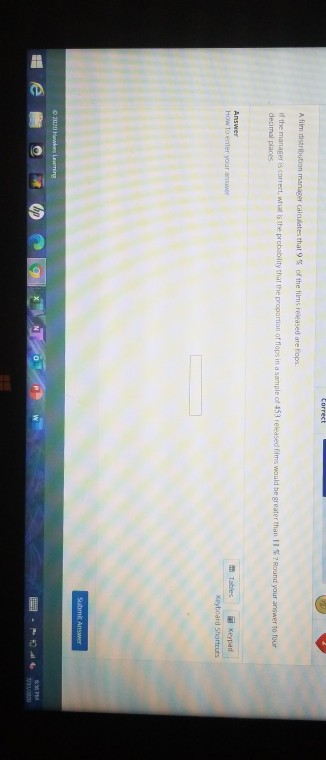Correct A film distribution manager calculates that of the films released are flops if the manager is correct, what is the probability that the proportion of flops in a sample of 453 released films would be greater than 117 Round your answer to four decimal places Answer How to enter your answer Tables Keypad Keyboard Shortcuts Submit Answer women e SPM VO

• ### A hotel manager calculates that 10% of the hotel rooms are booked. If the manager is...

A hotel manager calculates that 10% of the hotel rooms are booked. If the manager is correct , what is the probability that the proportion of rooms booked in a sample of 433 rooms would be less than 7%. round your answer to four decimal places.

• ### A bottle maker assumes that 18% of his bottles are defective. If the bottle maker is...

A bottle maker assumes that 18% of his bottles are defective. If the bottle maker is accurate, what is the probability that the proportion of defective bottles in a sample of 626 bottles would differ from the population proportion by more than 4%? Round your answer to four decimal places.

• ### Suppose a large shipment of stereos contained 21% defectives. If a sample of size 348 is...

Suppose a large shipment of stereos contained 21% defectives. If a sample of size 348 is selected, what is the probability that the sample proportion will differ from the population proportion by more than 4%? Round your answer to four decimal places.

• ### The quality control manager at a computer manufacturing company believes that the mean life of a...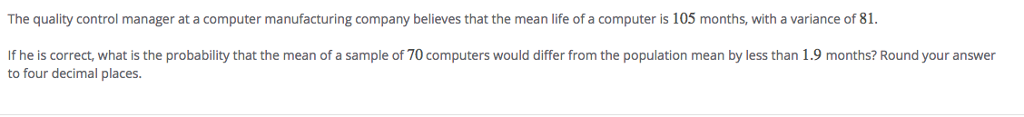The quality control manager at a computer manufacturing company believes that the mean life of a computer is 105 months, with a variance of 81 If he is correct, what is the probability that the mean of a sample of 70 computers would differ from the population mean by less than 1.9 months? Round your answer to four decimal places.

• ### The operation manager at a tire manufacturing company believes that the mean mileage of a tire...

The operation manager at a tire manufacturing company believes that the mean mileage of a tire is 49,36049,360 miles, with a variance of 6,568,9696,568,969. What is the probability that the sample mean would differ from the population mean by less than 231231 miles in a sample of 247247 tires if the manager is correct? Round your answer to four decimal places. CAN YOU PLEASE SHOW ME HOW TO DO THIS IN CALCULATOR TI-83 PLUS SPECFICALLY

• ### 56% of all newly released films in theaters are animated movies. But just because a film...

56% of all newly released films in theaters are animated movies. But just because a film is animated doesn’t mean it’s necessarily suitable for children. Of these animated movies, 29% of them are rated R. For all non-animated movies, 70% are rated R. (It may be helpful to use a probability tree to answer the questions below.) Use a probability tree to answer the following questions: (Round all answers to four decimals) a. A new movie was just released in...